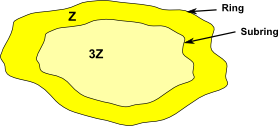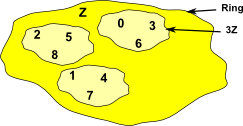# Maths - Ring

A ring is a rich and interesting mathematical structure. It is important, not least because it is the structure of our most common algebras such as the integers.

A ring is a concept similar to a group, that is a set together with an operation on that set, except that a ring has two operations.

These two operations are represented by addition and multiplication.

What makes a ring interesting, that is more than just two groups glued together, is the way the two operations interact with each other. This interaction between the two operations is defined by the distributive laws.### Axioms

associativity (a+b)+c=a+(b+c) (a*b)*c=a*(b*c)
commutativity a+b=b+a not required (see field)
distributivity     a*(b+c)=a*b+a*c
(a+b)*c=a*c+b*c
identity a+0 = a
0+a = a
not required (see field)
inverses a+(-a) = 0
(-a)+a = 0
not required (see field)

Note: it is not necessary that multiplication commutes or has a identity element or inverse. For that we mode to a more specific type of field known as a 'field', see this page.

Why do these particular rules produce such an interesting and important mathematical structure? The theory of rings seems less orderly than the theory of groups and some of the concepts seem to come out of nowhere and I find it hard to see why a particular concept has been used rather than some other.

The best answer I can come up with goes along these lines: with an additive group the generator <x> produces the additive part of the integers, if we imagine a similar generator <x> on a ring, that is we generate every combination of + and * on 'x' then we get a polynomial:

a0+ a1 x + a2 x2+ a3 x3+ a4 x4...

### IdealsA is ideal if, for every rR and every aA,

• ra is in A (right ideal)
• ar is in A (left ideal)
• ra & ar is in A (two sided deal)

i.e. A 'absorbs' elements from R

### Generating Ideals

<a> = { ra | rR} = an ideal of R called the principle ideal generated by a.
<a1,a2…an> = { r1a1,r2a2…rnan | riR} = an ideal of R generated by a1,a2…an.

So an ideal must be defined relative to some larger ring. We cannot just define <a> on its own without specifying this container.

For instance this containing ring may be:

1.,Q,Z,N: The reals, rationals, integers and so on.
2. 3Z, Z3: Some subset of the above.
3.[x],Z[x]: The set of all polynomials with, say, real or integer coefficients.
4. Any other ring, possibly a finite ring.

### Test for being an Ideal

1. a-bA whenever a,bA
2. ra and ar are in A whenever aA, rR.

### Example 1

Ideal Containing Ring
<3> Z<3> = {3r | rZ}=3Z

where 3Z = 0,3,6…

Since 3Z is a multiple of 3, when multiplied by an integer it is still a multiple of 3.

### Example 2

Ideal Containing Ring
<x>[x][x] = set of all polynomials with real coefficients
<x> = set of all polynomials with constant term = 0

### Factor Rings

R/A = { r + A| rR} = Factor Group (set of cosets).#### Example 3

Z/3Z = { r + 3Z| rZ} = { 0 + 3Z,1 + 3Z,2 + 3Z}= modulo 3 arithmeticZ …-2,-1,0,1,2… 3Z …-6,-3,0,3,6… 3Z+1 …-5,-2,0,4,7… 3Z+2 …-4,-1,0,5,8…

### Notation

notation description
Q[x] The set of all polynomials in the variable x with rational coefficients.[x] The set of all polynomials in the variable x with real coefficients.
Q[x]/<x²+1>

The smallest structure (field) that contains Q and also has a root of the polynomial x²+1.

The elements of Q[x]/<x²+1> are not polynomials but equivalence classes of polynomials.

<a>

an ideal of R called the principle ideal

{ra | rR } R is a commutative ring with unity

Q(√2) The smallest field outside Q that contains √2 (the splitting field)
Q(√2,√3) The smallest field outside Q that contains √2 and √3 (the splitting field)
C=(i)
=[x]/<x²+1>
The complex numbers are the smallest extension of the field of real numbers which includes i (or i is adjoined to)

[Q(√2):Q]=2
[Q(√2,√3):Q]=4
[C:]=2

degree of the extension. This is the number of terms required for a general element such as: a + b√2
p(x) a polynomial over x

Where I can, I have put links to Amazon for books that are relevant to the subject, click on the appropriate country flag to get more details of the book or to buy it from them.The Princeton Companion to Mathematics - This is a big book that attempts to give a wide overview of the whole of mathematics, inevitably there are many things missing, but it gives a good insight into the history, concepts, branches, theorems and wider perspective of mathematics. It is well written and, if you are interested in maths, this is the type of book where you can open a page at random and find something interesting to read. To some extent it can be used as a reference book, although it doesn't have tables of formula for trig functions and so on, but where it is most useful is when you want to read about various topics to find out which topics are interesting and relevant to you.